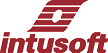Intusoft technical literature and publications

Texas Instrument
Getting Started
Lessons
Code Generator
Project, zip file with code and drawings

Microchip
Getting Started
Lessons
Code Generator
Project, zip file with code and drawings

Solar2TiM Eval Board
Drawing,  Layout,  BOM

DSP Designer is used for development of control systems implemented using digital signal processors. There are 5 components:

 1. Hardware evaluation kit 2. IntuScope, "DSP Communication Filter" plug-in which is used for real time DSP control and data acquisition. Real time communication, RTCOM, is used to perform AC, DC and TRAN analysis within the target DSP. 3. DSP Code Generators 4. New ICAP/4 Models and " DSP" analysis 5.C and Assembly code Template Projects

The optional hardware evaluation kit is designed to get you started with a real-world design.

These components are discussed in detail below.

Hardware Option
You can purchase 1 of 2 variations (or both), targeted for commercially available DSP's.

 1. Microchip DSPIC33FJ16GS502-I/SP, includes the Microchip 16-bit x 28-pin Starter with the DSP installed 2. Texas Instruments TMS320F28027 Piccolo DSP includes the Piccolo MCU controlSTICK evaluation Tool.

The hardware option includes 1 Intusoft Solar2TiM dual synchronous buck/boost PWM regulator with connectors that accept either of the above DSP evaluation kits.

Other DSP's can be plugged into the Solar2TiM connectors by wiring mating connectors to the user breadboard area of an evaluation board such as the Analog Devices Adu7026 Eval Board.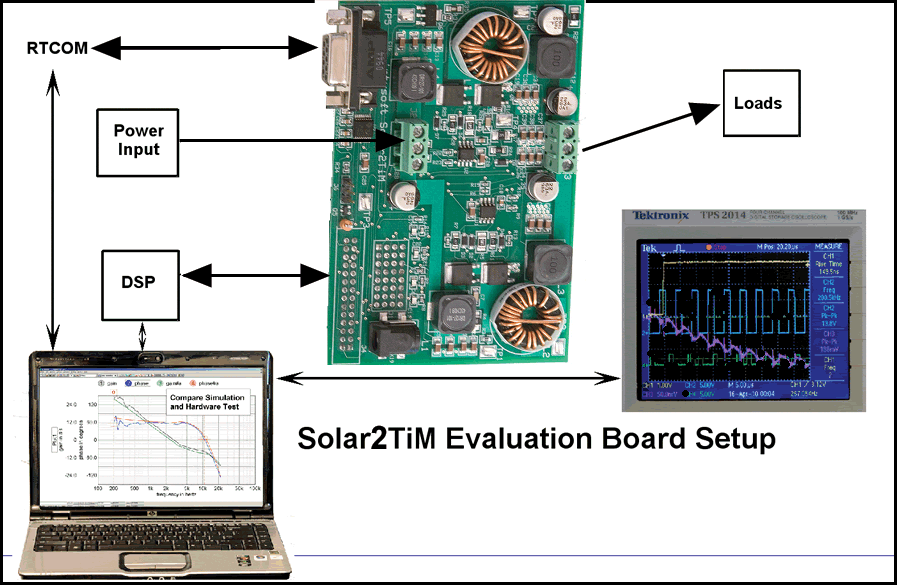RTCOM

Real time communication uses a small footprint DSP based UART communication via an RS232 or USB interface. Communication is achieved by reading and writing to a 8-word billboard region of RAM, thereby eliminating the possibility of changing critical RAM or I/O locations. Code in the DSP interprets the data written as one of 3 command types: AC, DC, and TRAN. These are hardware counterparts to a SPICE simulation.

RTCOM connects to the DSP using an IntuScope interface. IntuScope reads the DSP map file in order to find the addresses of variables. A corresponding ".dspt" file contains these addresses along with user supplied scale factors. In a new IntuScope feature, the op analysis vectors are now available in the "Add Waveforms" dialog vector list. Applying that to DSP communications allows you to read vector values defined in the DSP Communication Setup dialog and also to write data into the 8 word billboard. Pressing the Read button displays the vector value in the output window. Scripts are used to assign values to billboard locations and read data from the DSP.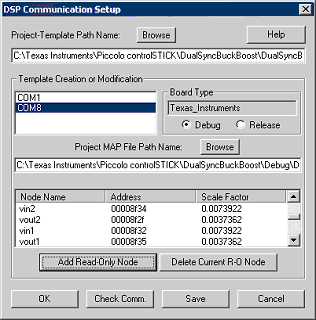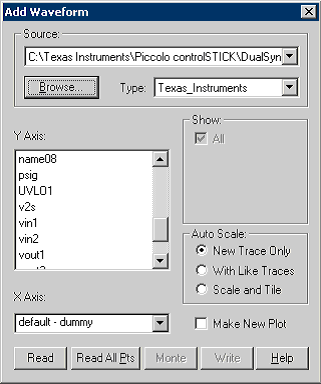AC

The hardware based AC analysis is a swept frequency transfer function analyzer based on single injection GFT theory. Running in about 20 seconds, it collects about 50 data points, from 200Hz to about .3*(Fs, the sampling frequency), as shown in the graphic to the right. The DSP generates a sine-cosine waveform algorithmically using much less memory than a table generated waveform generator. The cosine wave is scaled and inserted in series with the error signal. The coefficients for the waveform generator are transferred using RTCOM and the DSP waits for transient residues to stabilize, then it sums the product of cosine and sine times the signal. After 50ms of real time, it returns the result to IntuScope. IntuScope then computes the gain and phase for that frequency and advance to the next frequency.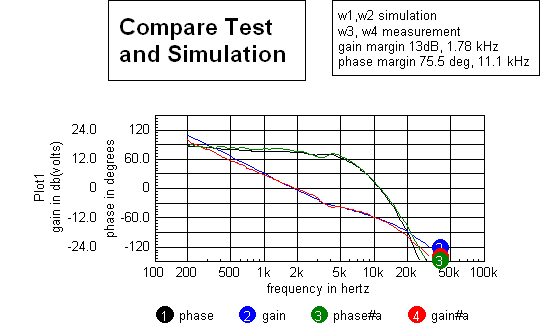DC

This corresponds to a SPICE DC operating point. It reads the steady state average of 1024 successive measurements of the specified vector. The IntuScope "Add Waveforms" dialog contains the standard 8-word interface along with user specified data and scaling. When SpiceNet drawing test points have the same name as the "Add Waveform" vectors, then SpiceNet can display the values by selecting "options/Stream DSP Testpoint Values" as shown in the graphic to the right. Averaging decreases noise by the square root of the number of samples; in this case, the virtual current estimate is 10ma RMS, which is equivalent to 8.7 bit accuracy.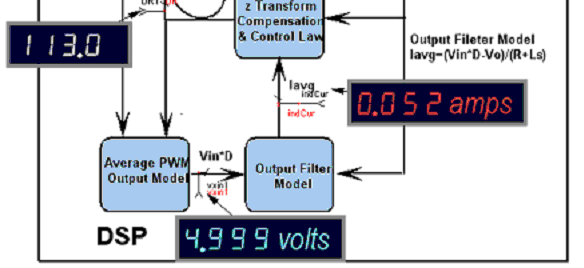TRAN

Lets you see the response of a named vector (from the "Add Waveforms" dialog) to a transient signal synchronized to TP5. TP5 drives an onboard MOSFET switch that can be connected externally to a load resistor. For each high-speed interrupt, one data point is added to the vector. Then the time slot in the vector for the data point is advanced by one sample. The built-in TRAN function takes 32 data points, averaged for 8 entire waveforms. It takes about 30 seconds to acquire the waveform. The internal error signal is plotted in the graphic to the right. This information is ONLY available in simulation or by using RTCOM because it is not available using hardware measurement because it is a mathematical representation inside of the DSP code.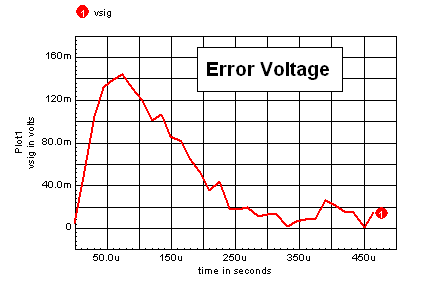_______________________Code Generation_______________________
Schematic: First, the user makes a schematic diagram of the controller in a separate drawing configuration.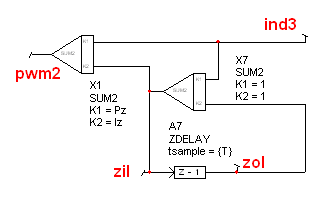PI Feedback Controller

IsSpice4 Matrix: Then, the ICAP/4 simulator builds a matrix that solves for the states shown as nodes in the schematic. Parameters define the variables so that the design becomes a template which applies to design variations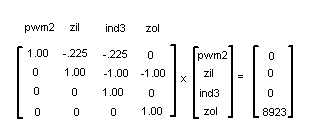IsSpice, ".DSP" Matrix

Sample of Assembly Code:

sl w2, #2 , w2  ; Scale ADC voltage
mov #1343, w1   ; get ref
sub w1, w2 ,w0  ; (ref - ADC) -> w0

; ####### Limit w0 for ind3
...

; PWM output for pwm2
mov w0 , PDC2
; propagate states
; PIcontroller_Statei.zoi = _Statei.zii;    mov [w8+2], w0
mov w0, [w8+6]

 _______________ New ICAP/4 Models and Analysis________________
 Z-Transform Theory

DSP controllers use Z-Transforms to express a control law. Z-Transform transfer functions can be mapped from the continuous or Laplace domain to the Z plane by evaluation the defining equation: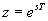, where s is the Laplace operator and T is the sample period. Solving for s and using a truncated series expansion yields several ways to define the process of ntegration.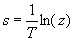The ln(z) function can be broken down into several common approximations. Let's first do this by using the first term of the series expansion yielding the bilinear transformation: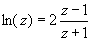In the frequency domain,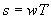, then
for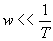then we can approximate the denominator as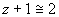, and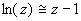Then solving for the integral 1/s yields,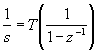The Direct programming method resolves this equation into the following block diagram: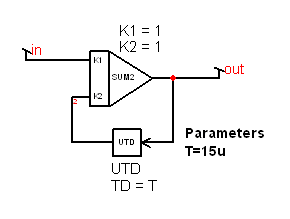This is the same equation that can be derived using forward Euler integration

Control expressions can be written using the definition of integration and its inverse, differentiation directly. The classical PID (proportional integral differential) controller is a widely used example. It is one of the template designs included in the DSP Designer projects.

Another controller approach proposed by Meares computes the switched inductor current based on observed measurements and uses that "virtual current" as the input to an average current controller. Using virtual current eliminated the need for current measurement that's especially difficult for bi-directional power transfer associated with synchronous switching. Current feedback provides improved damping of the L-C filter resonance compared to a PID controller. Moreover, the PID controller pole-zero canceling breaks down in the presence of the input filter.

 ZDELAY

Typically, SPICE simulators use the transmission line to model the unit delaywith delay = T. But Z-Transform theory is limited to the study of a sampled signal at the sampling instants. The transmission line, on the other hand, is a continuous device that has values in between sampling instants. In fact, it's perfectly permissible for a Z-Transform solution to oscillate at frequencies above the sampling frequency. That can cause the SPICE simulation to spend extra time in its transient simulation generating unwanted data. Intusoft has introduced a new model called ZDELAY. It behaves the same as a transmission line for AC analysis calculation, but in the transient analysis, it samples the data every T seconds and sends the sampled data to its output after a specified computational delay. When viewed with an IntuScope probe, the result looks like a zero order hold as shown with a sine wave input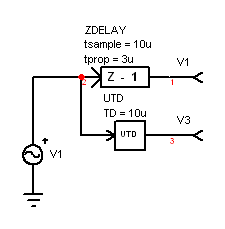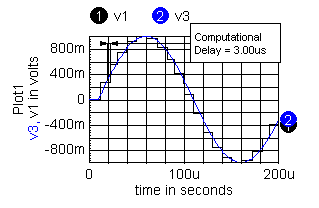Data in between samples uses the input following the computational delay. If many ZDELAY elements are used, there is only one computational delay which is effectively in series with the output. That effect is not included in the AC analysis, so you must add an extra ZDELAY element is series with the output D/A conversion for AC analysis only.

 .DSP Analysis

The SPICE transient simulation proceeds by solving a modified nodal admittance matrix, MNA. It iterates the solution at each time point until its convergence criteria is met. This method is required for solving non-linear equations. But difference equations for a DSP controller can be made to be linear. Imposing a linear constraint can result in an upper diagonal matrix (values below the main diagonal are zero) that is used over and over for each DSP sample time. If the matrix is ordered so that unwanted states are at the top and trivial states at the bottom, then solution by backward substitution from the fist non-trivial state to the first unwanted state produces a solution in a single pass. Trivial states are identified as having just one non-zero state in the matrix. For a linear matrix, the right hand side, RHS, is zero for each non-trivial state. The new ".DSP" analysis follows these rules. After the solution is made, the solution for the ZDELAY inputs are propagated to their outputs before the next sample, and are among the trivial states. Other trivial states are measured inputs and outputs from other control algorithms. The resulting matrix can be seen in the ".out" file.

This method works well for the PID controller; however, the virtual current controller must estimate current that is a function of duty ratio, input voltage and output voltage in order to compute the duty ratio, A linear approximation is made by using the previous duty ratio to compute the average current, thus breaking up the non-linear portion of the control law. The effect of doing this can be simulated and as it turns out doesn't have much effect of the controller. It should be noted that the controller could be allowed to iterate the solution; however, a stable result can't be guaranteed and the state matrix would have to be re-computed for each iteration. The former constraint is untenable for a real time control system.An interesting results of configuring a linear DSP controller is that the DC operating point is the solution of a set of independent linear equations that always must have a unique solution. But the down side is that the user must supply the correct ZDELAY initial conditions. Running a transient solution to steady state yields the IC's needed for the AC analysis. The user needs some insight in the design of the controller so that it has a stable steady state solution. Compensating the control transfer function for gain and phase margin can then proceed.